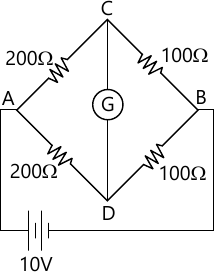MORE IN Electronic Instrumentation
VTU Electronics and Communication Engineering (Semester 3)
Electronic Instrumentation
December 2014
Total marks: --
Total time: --
INSTRUCTIONS
(1) Assume appropriate data and state your reasons
(2) Marks are given to the right of every question
(3) Draw neat diagrams wherever necessary

1(a) Define the following terms as applied to an electronic instruments:
i) Accuracy ii) Significant figure iii) Resolution.
6 M
1(b) Draw a basic DC voltmeter circuit,derive expression for multiplier resistance and calculate its value for a voltage range of (0-10)V if a full scale deflection current of 40?A and internal resistance of the meter is 500?.
8 M
1(c) Explain the working of a true RMS voltmeter with the help of a suitable block diagram
6 M

2(a) Explain the working of linear ramp type DVM.
8 M
2(b) Explain the working of a digital frequency meter with the help of a block diagram.
8 M
2(c) What is 3-1/2 digit DVM? Define its sensitivity.
4 M

3(a) Draw the basic block diagram of an oscilloscope. Explain the function of each block.
8 M
3(b) Describe the following modes of operation in a dual trace oscilloscope:
i) alternate mode ii) Chop mode
8 M
3(c) What is the role of Time base generator?
4 M

4(a) Explain the operation of a digital storage oscilloscope with the help of a block diagram. Mention the advantages.
10 M
4(b) Explain Mesh storage and phosphor storage techniques in storage oscilloscope.
6 M
4 M

5(a) What is Barkhausen criteria? Explain with block diagram AF sine-square wave generator.
10 M
5(b) Explain general pulse characteristic.
4 M
5(c) Explain working sweep frequency generator.
6 M

6(a) The Wheatstone bridge is shown in fig.Q6(a) below.The galvanometer has a current sensitivity of 12mm/uA. The internal resistance of galvanometer is 200?, Calculate the deflection of galvanometer cased due to 5? unbalance in the arm AD.6 M
6(b) Explain and derive expression for Maxwell's bridge constants are $C_{1}-0.5\mu f,R-1200\Omega ,R_{2}-700\Omega,R_{3}=300\Omega.$
8 M
6(c) Explain Wagner's Earth connection
6 M

7(a) Explain Resistive position Transducer and a resistance position traducer user a shaft with a stroke of 3 inch. The total resistance of the potentiometer is 5\Omega,. Calculate the output voltage when wiper is 0.9 inch form extreme end if applied voltage is 5V.
8 M
7(b) Explain construction,principle and operation of LVDT. Show characteristics curves.
6 M
7(c) What is thermistor? Explain different Forms of thermistors.
6 M

8(a) Explain in brief effects of photo conductive and photovoltaic transducer.
6 M
8(b) What is LED and I.C.D in brief.
6 M
8(c) Write short notes on :
i) RF Power Measurement using Bolometer
ii) Lab view
8 M

More question papers from Electronic Instrumentation